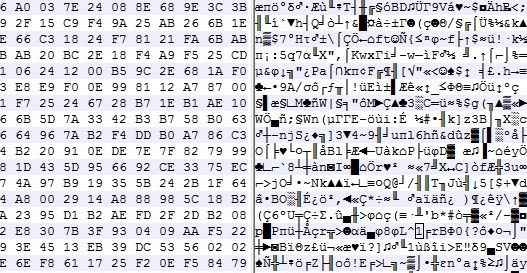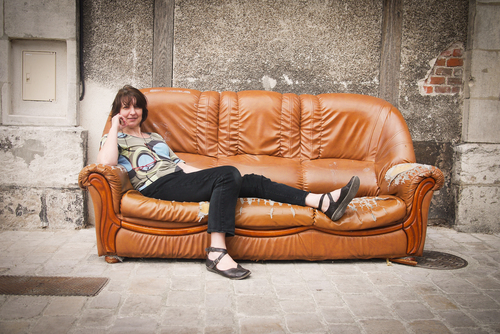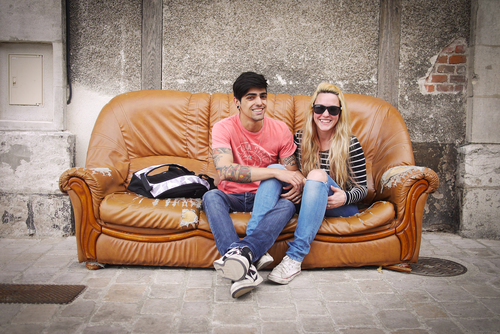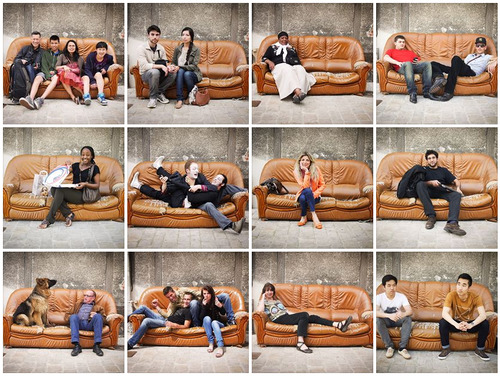28/09/2023

# #random

## An attempt to generate random data with audio (and your computer's built-in microphone)You probably know that generating some real random data is not so easy to do with a computer. How to design a good Random Number Generator (or a pseudo-random one) is a math topic that you can work years on ; it's also something very important for real-life applications such as security/cryptography, for example when you need to generate strong passwords.

Usually (and this is true in general in cryptography), designing your own algorithm is bad, because unless you're a professional in this subject and your algorithm has been approved by peers, you're guaranteed to have flaws in it, that could be exploited.

But here, for fun (don't use it for critical applications!), let's try to generate 100 MB of good random data.

1) Record 20 minutes of audio in 96khz 16bit mono with your computer's built-in microphone. Try to set the mic input level so that the average volume is neither 0 dB (saturation) nor -60 dB (too quiet). Something around -10 dB looks good. What kind of audio should you record? Nothing special, just the noise in your room is ok. You will get around 20*60*96000*2 ~ 220 MB of data. In these 220 MB, only the half will be really useful (because many values in the signal - an array of 16-bit integers - won't use the full 16-bit amplitude: many integers "encoding" the signal might be for example of absolute value < 1024, i.e. will provide only 10 bits)

2) Now let's shuffle these millions of bits of data with some Python code:

from scipy.io import wavfile
import numpy as np
import functools

#### GET A LIST OF ALL THE BITS
L = []  # list of bits
for i in range(len(x)):
bits = format(abs(x[i]), "b")  # get binary representation of the data
# don't use "016b" format because it would create a bias: small integers (those not using
# the full bit 16-bit amplitude) would have many leading 0s!
L += map(int, bits)[1:]        # discard the first bit, which is always 1!

print L.count(1)
print L.count(0)  # check if it's equidistributed in 0s and 1s

n = 2 ** int(np.log2(len(L)))
L = L[:n]  # crop the array of bits so that the length is a power of 2; well the only requirement is that len(L) is coprime with p (see below)

### RECREATE A NEW BINARY FILE WITH ALL THESE BITS (SHUFFLED)
# The trick is: don't use **consecutive bits**, as it would recreate something close to the input audio data.
# Let's take one bit every 96263 bits instead! Why 96263? Because it's a prime number, then we are guaranteed that
# 0 * 96263 mod n, 1 * 96263 mod n, 2 * 96263 mod n, ..., (n-1) * 96263 mod n will cover [0, 1, ..., n-1].  (**)
# This is true since 96263 is coprime with n. In math language: 96253 is a "generator" of (Z/nZ, +).

p = 96263  # The higher this prime number, the better the shuffling of the bits!
# If you have at least one minute of audio, you have at least 45 millions of useful bits already,
# so you could take p = 41716139 (just a random prime number I like around 40M)

M = set()
with open('random.raw', 'wb') as f:
for i in range(0, n, 8):
M.update(set([(k * p) % n for k in range(i, i+8)]))  # this is optional, here just to prove that our math claim (**) is true
c = [L[(k * p) % n] for k in range(i, i+8)]   # take 8 bits, in shuffled order
char = chr(functools.reduce(lambda a, b: a * 2 + b, c))  # create a char with it
f.write(char)

print M  == set(range(n))  # True, this shows that the assertion (**) before is true. Math rulez!

Done, your random.raw file is filled with random data!

Notes:

• The only issue I can see happen right now is if the ADC (analog-to-digital-converter) electronic component of your soundchip is highly biased (please drop me a message if you have such a device).

• This code here is unoptimized, it took 2 minutes for 1 minute of audio. There's surely a better way to work with arrays of bits in Python, comments/improvements are welcome!

• How to test the randomness quality of this file? This is a complicated task, and here are some references to do that. This is very far from being a rigorous way to do it, but it can be a first step (quote from the linked page): I've seen winzip used as a tool to measure the randomness of a file of values before (obviously, the smaller it can compress the file the less random it is). If you do it on the file generated here, you get exactly the same size (or even a bit more) after zip-compressing the file! Idem with rar, 7z (which usually yield a far better compression ratio, especially for audio data), the compression ratio is 1:1.

## On random multiplicative functions

Let's consider a sequence $(f(p))_{p \ prime}$ of independent random variables taking values ±1 with probability 1/2, and extend $f$ to a multiplicative arithmetic function defined on the squarefree integers.

Finding an upper bound for $M(x) = \sum_{n \leq x} f(x)$ has been long studied. Wintner proved in 1944 that $M(x) \ll x^{1/2 + \varepsilon}$ a.e., later improved by Erdös who establishes $M(x) \ll \sqrt{x} (\log x)^c$. Halász then obtains in 1983 the upper bound $M(x) \ll \sqrt{x} e^{c \sqrt{(\log\log x)(\log\log \log x)}}$. In a preliminary work by Lau, Tenenbaum, Wu, the bound $M(x) \ll \sqrt{x} (\log \log x)^{5/2 + \varepsilon}$ has been obtained.

With the use of martingale methods (new in this context at this time), a generalization of the Doob inequality (Hájek-Renyi inequality) and other techniques, I improved this bound to:

$$M(x) \ll \sqrt{x} (\log \log x)^{2 + \varepsilon} \qquad\textrm{a.e.}$$

This was the goal of my work Sommes friables de fonctions multiplicatives aléatoires published in Acta Arith., 2012, as well as obtaining estimations of the type $\Psi_f(x,y)\ll \Psi(x,y)^{1/2+\varepsilon}$ on y-smooth (a.k.a. friable) integers ≤ x.

Is it possible to improve the exponent $2+\varepsilon$ further?
The question remains open (the exponent $3/2 + \varepsilon$ had been claimed in a paper – but then removed in an updated version).

## Un Canapé à Orléans – Expérience photographique aléatoire

Un jour de 2013, je passe à côté d'un vieux canapé, abandonné, au coin de la rue d'Alibert. J'étais dans une période résolument "photographique" : en tant que nouvel orléanais, quoi de mieux que la Street Photography pour découvrir une ville et ses habitants ? Je décide donc de chercher mon appareil, et dix minutes plus tard, me voilà installé à attendre les passants et leur suggérer de prendre la pose (pause ?) sur ce canapé.

A ma grande surprise, les personnes étaient majoritairement volontaires pour se préter au jeu. La question "Mais dans quel but ?" avait pour réponse "Simplement pour une photo, et éventuellement pour une expo", chose qui fut réalisée au festival Les Ingrédients (Ingré) l'année suivante et au bar L'Escargot (Place de Loire, Orléans).

Au fil des jours, je déposais donc les nouveaux clichés sur la page Un Canapé A Orléans, et de nouvelles personnes arrivaient – soit de simples passants ou des gens qui avaient vu la page sur internet.

Merci aux personnes du service voirie de la ville qui ont accepté de retarder l'enlèvement du-dit canapé de quelques jours, à la République du Centre pour leurs articles, à la parution Publicités sur canapé du magazine Arrêt sur Images, et bien sûr, à toutes les personnes photographiées!My personal blog.

Data / AI / Python consulting and freelancing.